## Learning Outcomes

Aids to Understanding

Since linear velocities and accelerations, our usual terms of reference in kinematics, are defined in terms of displacement we need to define displacement in a circular context. We will call this angular displacement, q. It is clear that if a body travels round 1/8 circle, it subtends an angle of 45o, where 1o is 1/360 th part of a circle. Although this is perfectly fine for many purposes we approach angular measurement differently. We note that the length of an arc, s, is proportional to the angle subtended therefore it makes sense to define angular displacements, q, as the ratio of arc length to radius; q = s/r. Thus 45o is equivalent to a dimensionless number that has a value of p/4. And we say 45op/4 radians (rad). Since 2pr is the full circular arc (or circumference) and a body covering this length subtends an angle of 3600 it should be clear that 2p rad ≡ 360o and p/4 rad ≡ 45o.

Click for homework on Rotational Motion Try to have this completed for the Tuesday 27th September after Rome.

## 1) Rotational kinematic relationships as derivatives

In the previous topic we discussed the equations of motion in derivitave form. The motion was linear. In this topic we discuss rotational motion and express the equations of motion in terms of angular quantities. By rotational motion we mean bodies travelling specifically in a circle.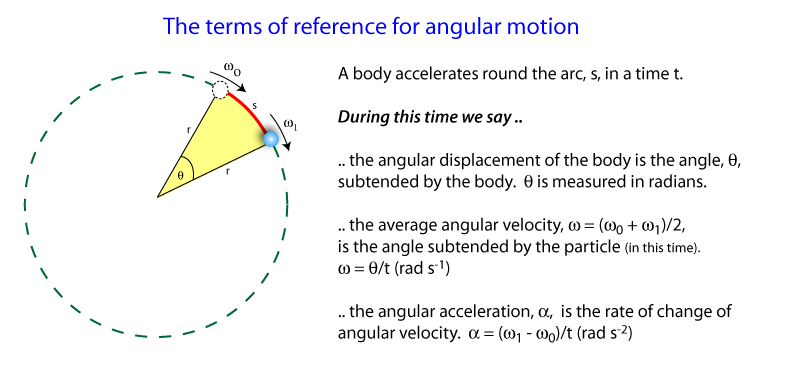## 2) Angular acceleration

For linear motion we defined acceleration and velocity as time dependent derivatives. For rotational motion we also define our terms as time dependent derivatives. This leads to analogous equations of motion for rotational quantities. As you can see the angular acceleration is defined as the rate of change ofangular velocity and angular velocity is defined as the rate of change of angular dsplacement.## 3) The rotational equations of motion

The equations of linear and angular motion are presented below. It should be clear that questions that involved linear motion are exactly the same in principle with questions that involve rotating bodies.
Equations of linear motion Equations of rotational motion
s= ut + 1/2at2 q = wot + 1/2at2
v= u + at w = wo + at
v2 = u2 +2as w2 = wo2 + 2aq

## 4) Typical rotational motion problem

The following example illustrates a context for the equations of rotational motion.
Example: A helicopter is flying through the air at a steady speed and height. The blades are rotating at 250 revs/min. The pilot accelerates for 10s, and the helicopter's blades increase their rotation to 350 revs/min.
1. Calculate wo the initial angular velocity
2. Calculate w1 after 10 s
3. Calculate a,the angular acceleration
4. Calculate q the angular displacement of the blades during the 10 s.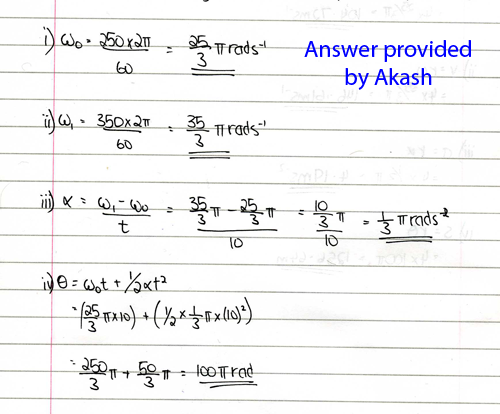## 5) Tangential and Angular velocities

Although rotational quantities are important it is also useful to find the tangential speed and acceleration of the body as it traverses a circle. The tangential speed is measured in ms-1. If we assume arc length s = distance, then s = rq becomes d = rq. So v=d/t becomes v = rq/t = rw, ie v = rw.

## 6) Tangential and Angular acceleration

The tangential acceleration is measured in ms-2. If a = (v-u)/t then a = (rw - rwo)/t
a = r(w - wo)/t
a = ra
This leads us to the following three expressions linking angular quantities to linear quantities.
• s = rq
• v = rw
• a = ra
1. Calculate u, the initial velocity of the blade's tip
2. Calculate v, the velocity of the blade's tip after 10 s.
3. Calculate a, the acceleration of the blade's tip.
4. Calculate s, the total displacement of the blade's tip during the 10 s acceleration.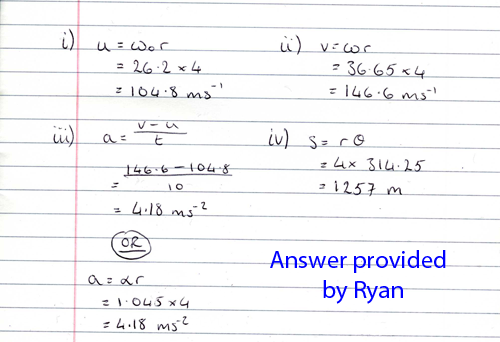## 7) Centripetal acceleration derivation

Whenever a body is travelling in a circular path it's direction is constantly changing therefore it is constantly accelerating. This acceleration is called the centripetal acceleration The magnitude of the acceleration depends on the magnitude of the body's velocity, v, and the radius of the circle (or curve), r, it is travelling around. The magnitude of the acceleration is v2/r and a derivation of this is provided by Ross Macgregor.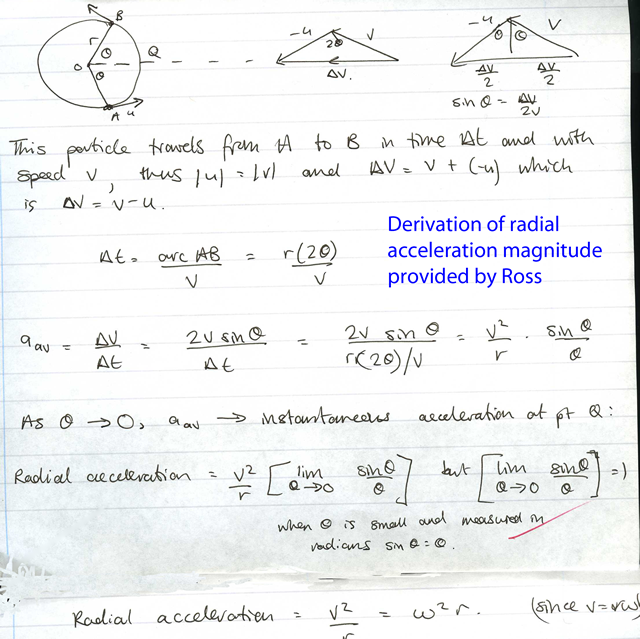Example Suppose a runner is running around a circular track of circumference 500 m. If the runner completes one complete lap in 100 s, calculate the runner's centripetal acceleration; Answer 20ms-2

If a body is accelerating then an unbalanced force acts on it. The unbalanced force on a body that causes a centripetal acceleration of a body is called a radial force. The central force is not a mysterious new force; it be frictional force, a tension or a weight depending on the context. The radial force is necessary to keep bodies in a circular path. The magnitude of the radial force, Fr, is equal to mv2/r and our challenge is to find the force from the context before we equate it to its numerical value of mv2/r. There are three motions to consider, horizontal motion, vertical motion and motion where the body is at angle to the horizontal.

Central force in horizontal context

(a) A car travelling around a bend As usual with all force problems we draw a free body diagram. Since the body is following a circular path it is accelerating therefore the free body diagram should show an unbalanced force. The unbalanced is the radial force.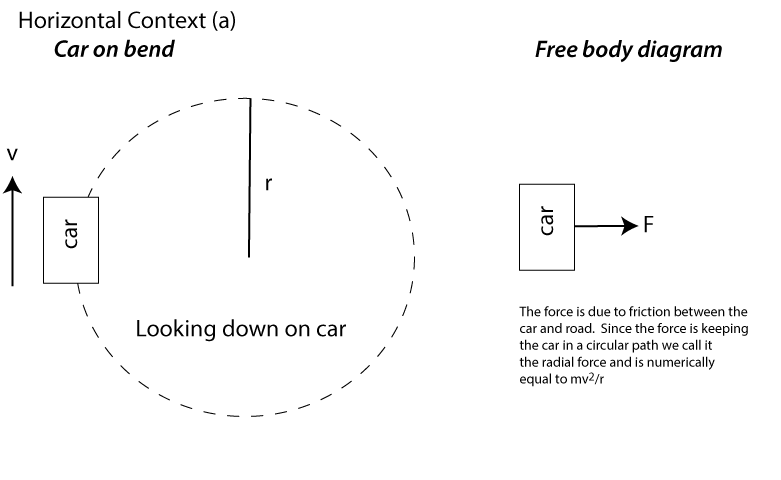(b) Body on string rotated in horizontal plane. As usual with all force problems we draw a free body diagram. Since the body is following a circular path it is accelerating therefore the free body diagram should show an unbalanced force. The unbalanced is the radial force.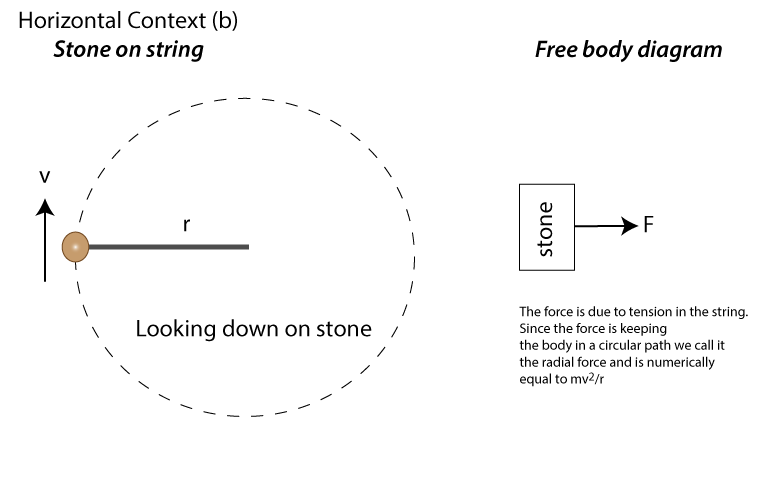Central force in vertical context

(a) Body on string rotated in vertical plane. The same principal applies as in the horizontal context, but now we must consider the body's weight.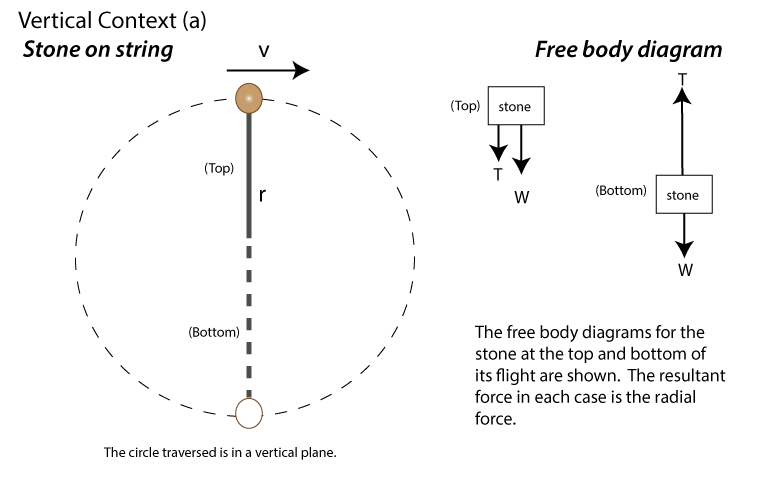Study this carefully and you should be able to see that the tension in the string is greatest at its lowest point of the flight. (b) Car on humped back bridge. In this context the forces acting on the car are its weight and the reaction force due to the bridge.If R = 0 then the car is not in contact with the bridge. The minimum speed of the car that meets this condition is the maximum speed the car can safely travel along the bridge without taking off. Rearranging mv2/r = mg for v will find this maximum speed. Radial force in angled context

The context is normally a car going around a banked track or a motorcyclist tilting over as it travels around a bend. In these contexts the radial force is the component of the bodies reaction force towards the center of the track.For this example it is the component of the reaction force, R, that constitutes the radial force. Note the vertical forces on the car are balanced but the forces towards the center (ie the radial force) is unbalanced. Look carefully at how these relationships are written above.

Example

A civil engineer wishes to bank a curve on a road so cars can travel around it with a maximum speed of 25 ms-1. If the curve a radius of curvature equal to 20 m, then calculate the banking angle required.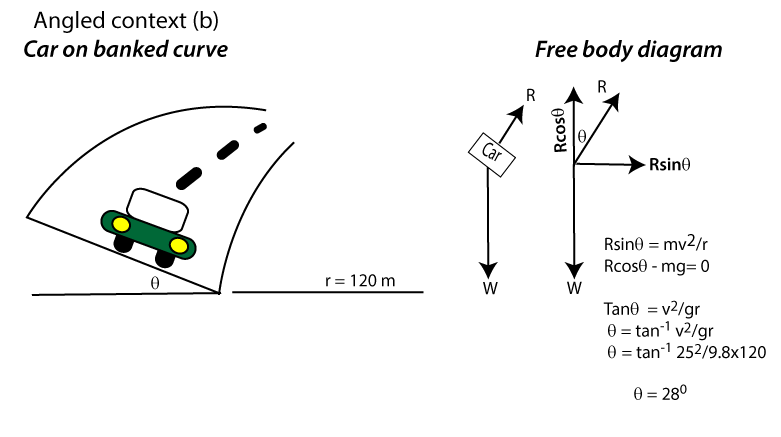The banking angle needs to be 28o. Notice that this angle does not depend on the mass of the car. Exceeding 25 ms-1 (90 mph) would result in the car leaving the road.